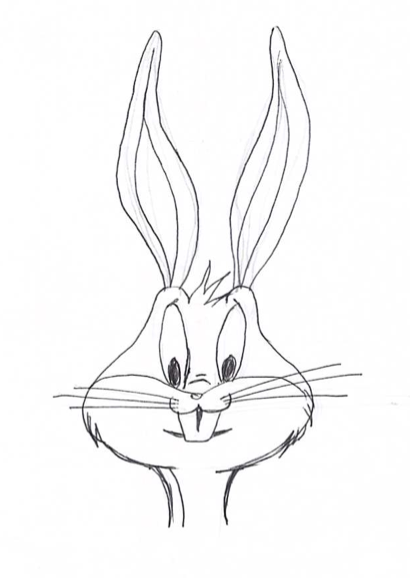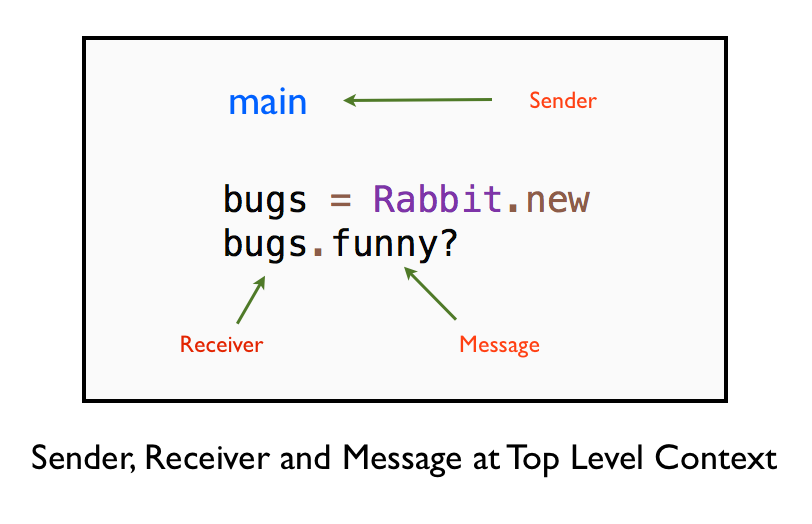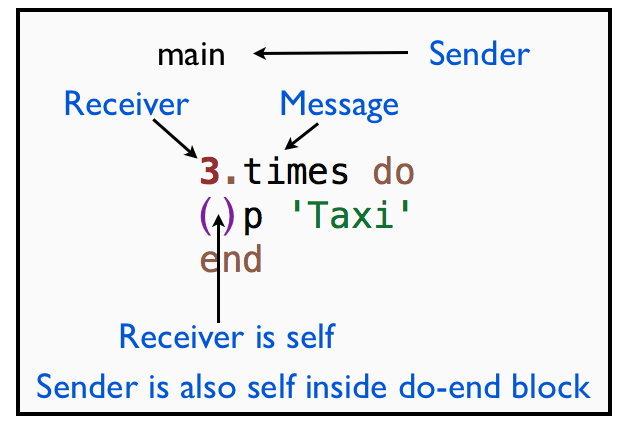# Message Sender at the Top Level

In this chapter you will learn that the main is the message sender when we call methods in the top level context.

## Main the Message Sender

Let's write a simple program to illustrate that main is the message sender at the top level.

``````class Rabbit
def funny?
true
end
end

bugs = Rabbit.new
p bugs.funny?
``````

This prints:

``````true
``````The message sender is the value of self, which is main in the top level context. The variable bugs is the message receiver and the message sent is funny?## Implicit Sender for Hail Taxi

Let's now find out the implicit sender for our simple hail taxi program. Where did the message originate? Message originates at:

``````3.times
``````

So, let's print the value of self before and after this line of code.

``````puts "outside loop self is #{self}"
3.times do
puts "inside loop self is #{self}"
p 'Taxi'
end
``````

This prints:

``````outside loop self is main
inside loop self is main
"Taxi"
inside loop self is main
"Taxi"
inside loop self is main
"Taxi"
``````

This demonstrates that main is the object that is sending the message. Thus, main is the sender. The self is acting as the default sender. The sender is implicit in the code, so it is invisible. But it is there in the context of executing the example hail taxi program. The Fixnum object 3 is the receiver and times is the message.Inside the do-end block, the sender and receiver are the same. Because the receiver of the p() and puts() methods is also main. This is subtle. If you understand this concept, you will have a solid foundation to become a Ruby expert.

## Implicit Sender for Teacher Program

In the Message Passing chapter, we saw the explicit sender for the teacher program. Let's take a look at that example so that we can identify the implicit sender.

``````class Teacher
def initialize(student)
@student = student
end

def ask_student_name
@student.ask_name
end
end

class Student
def initialize(name)
@name = name
end

def ask_name
@name
end
end

student = Student.new('Bugs Bunny')
teacher = Teacher.new(student)

p teacher.ask_student_name
``````

The implicit sender in this program is the main object. Look at the last line in the example. You can see that the teacher object is the receiver. The main sends the message ask_student_name to the teacher object. The reason is that at the top level, main is the sender object.## Fabio Asks

How do I identify the message sender?

Ask yourself the questions:

1. Where did the message originate?
2. Who is the owner of the scope where the message originated?

## Rhonda Asks

What is the message receiver in the teacher example?

You can ask Ruby:

``````def ask_student_name
puts "The sender object is : #{self.class}"
@student.tap do|x|
p "Before invoking ask_name, sender object is : #{self.class}"
p "Before invoking ask_name, receiver object is : #{x.class}"
end.ask_name
end
``````

The message receiver is student object.

## Summary

We saw an example for implicit sender where the sender object was implicit and hidden in the code. We also saw that implicit sender and implicit receiver are the same inside the do-end block for the hail taxi program. What is the use of knowing that the sender and receiver are the same? We will discuss this in the upcoming chapters on Same Sender and Receiver and Private Methods.